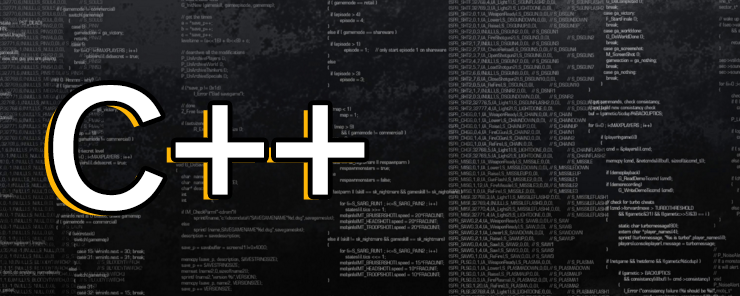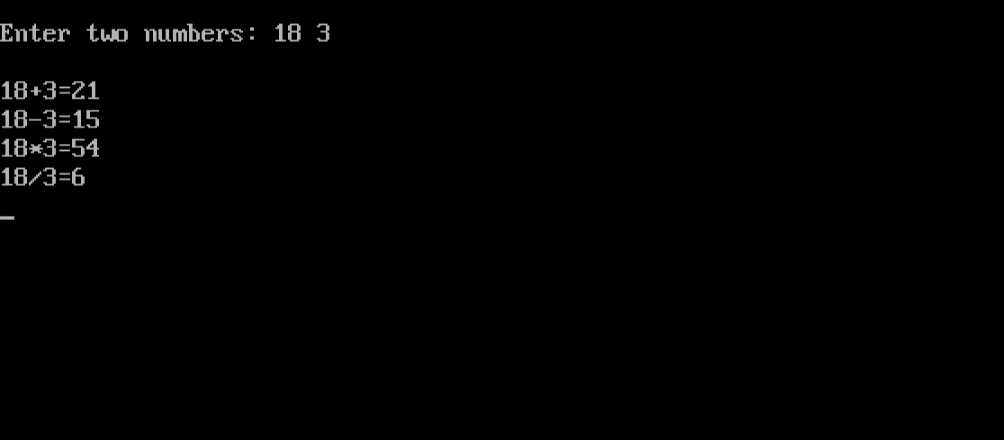# Learn about Operators in C++ Programming

0
2269After talking about Variables and Data Types, it is time to learn what to do with those variables. In this article, we are going to talk about Operators.

What are Operators?
For a programmer, operators are like the T-ruler for an architect. They are the tools that perform the required processing on data stored in variables.

C++ supports several types of operators. In the following sections, we are going to discuss them all, and illustrate their usage with examples.

Arithmetic Operators
To help you perform the basic arithmetic calculations, C++ provides a list of arithmetic operators:

 Operator Description + Adds the two operands. – Subtracts the right side operand from the left side one. * Multiplies the two operands. / Divides the left side operand by the right side one. % Divides the left side operand by the right side one, and returns the remainder.

Assignment Operators
Yes, there are assignment operators other than the normal assignment operator ‘=’. Consider the case when you need to calculate the total for a set of numbers. After initializing the total variable to 0, you start adding the numbers to the total variable, one after another.

```total=total+x1;
total=total+x2;
```

You will need to do this for every number to be added. C++ is nice enough to let you achieve this with less typing using the assignment operators.

 Operator Description += Adds the right side operand to the left side one, and stores the result in the left side operand. -= Subtracts the right side operand from the left side one, and stores the result in the left side operand. *= Multiplies the right side operand by the left side one, and stores the result in the left side operand. /= Divides the left side operand by the right side one, and stores the result in the left side operand. %= Divides the left side operand by the right side one, and stores the remainder in the left side operand.

We could use:

`Total+=x1;`

Which have the same meaning, but with less typing. Similarly, if you need to increment or decrement a counter, the following statement will do the job:

```counter+=1;
counter-=1;
```

Increment and Decrement Operators
For the special case of adding/subtracting 1 to/from a variable, C++ provides a shorter method (even shorter than the += and -= method). This is done using the increment and decrement operators (++ and –), respectively. So, to increment the counter variable, you could use the following statement:

```counter++;
or
++counter;
```

To decrement:

```counter--;
or
--counter;
```

Comparison Operators
Together with the Logical operators (will be discussed in the next section), the Comparison operators are essential for decision making. The following table lists the comparison operators supported in the C++ language:

 Operator Description < Returns true if the left side operand is less than the right side one. > Returns true if the left side operand is greater than the right side one. <= Returns true if the left side operand is less than or equal to the right side one. >= Returns true if the left side operand is greater than or equal to the right side one. == Equality operator. It returns true if both operands are equal. != Inequality operator. It returns true if the two operands are not equal.

Logical Operators
To combine two or more basic conditions into a more complex one, the Logical operators are used for this purpose. The logical operators are the software equivalence to what are known in logic as logic gates. The basic concept of the logic gates came from the machine circuitry. Consider two electrical switches connected together in series. For the current to flow from one point to another across the two switches, switch 1 AND switch 2 must be on. If they were connected in parallel, then switch 1 OR switch 2 needs to be on for the current to flow from one side to another. The NOT gate does nothing but inverting its input. This is the way I prefer to explain the logic gates concept.

C++ has three basic logical operators (equivalent to the basic logic gates: AND, OR, and NOT).

 Operator Description && Logical AND operator. It returns true if both conditions are true. || Logical OR operator. It returns true if any of the conditions (operands) is true. ! Logical NOT operator. Its output is the reverse of the input.

Example
We need to write a program that accepts two numbers from the user, and uses them to perform the basic arithmetic operations.

Consider the following code:

```#include<iostream.h>
#include<conio.h>
void main()
{
int a,b;
cout<<"Enter two numbers: ";
cin >> a >> b;
cout << endl;
cout << a << "+" << b << "=" << a+b << endl;
cout << a << "-" << b << "=" << a-b << endl;
cout << a << "*" << b << "=" << a*b << endl;
cout << a << "/" << b << "=" << a/b << endl;
getch();
}
```

Let’s see how this program will behave:Nice!!! Now, we need to explain some points:

• The strange #include<conio.h> statement in the 2nd line inserts another header file (conio.h) that is necessary for the getch() function to work without errors.
• The cin object can use several cascaded >> operators. This gives us the capability to accept more than one input using a single line of code. This is a short alternative to saying:
```cin>>a;
cin>>b;
```

It is obvious that cin>>a>>b; is shorter, and looks smarter as well.

• The getch() function gets a single character from the user. It reads the character directly from the keyboard without echoing it to the screen. It causes the program to wait (at the console screen) for the user to type something. Once it catches a keystroke, it returns. This function is usually used to keep the output screen (that displays the program output) until the user presses any keyboard key to close it.

* * * * * *

Summary

• C++ supports several types of operators: Arithmetic, Assignment, Increment, Decrement, Comparison, and Logical.
• Comparison and Logical operators are essential for decision making.

Now, we are ready for the next topic: Decision Making.
So, stay here, and don’t miss it!!